Finding Square root through prime factorisation

Chapter 5 Class 8 Squares and Square Roots
Concept wise

Let’s find square root of 36 using Prime factorization

First, we do prime factorization of 36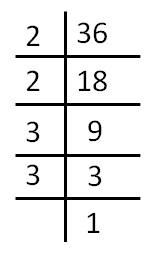36 = 2 × 2 × 3 × 3

Now,

we make groups of two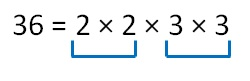So,

Square root of 36 = 2 × 3

= 6

#### Square root of 49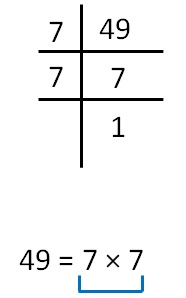So, Square root of 49 = 7

#### Square root of 64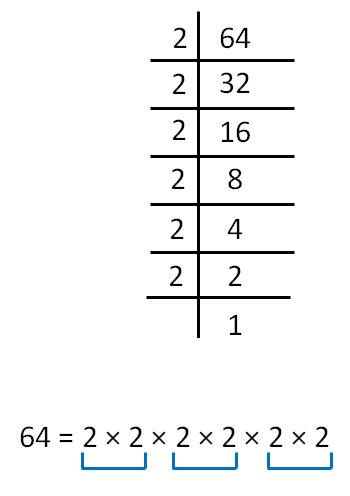So, Square root of 64 = 2 × 2 × 2

= 8

#### Square root of 324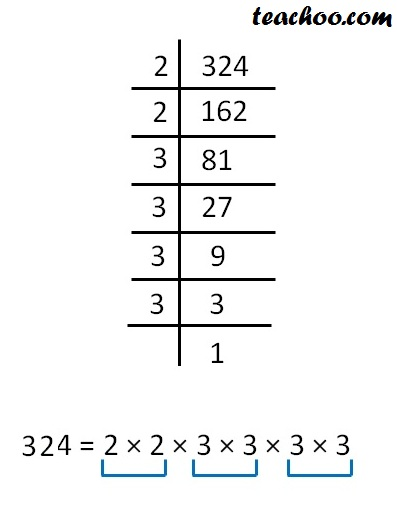So, Square root of 324 = 2 × 3 × 3

= 18

Learn in your speed, with individual attention - Teachoo Maths 1-on-1 Class